# 6.3 Solution and algorithm control

The equation solvers, tolerances and algorithms are controlled from the fvSolution dictionary in the system directory. Below is an example set of entries from the fvSolution dictionary required for the icoFoam solver.

17
18solvers
19{
20    p
21    {
22        solver          PCG;
23        preconditioner  DIC;
24        tolerance       1e-06;
25        relTol          0.05;
26    }
27
28    pFinal
29    {
30        \$p;
31        relTol          0;
32    }
33
34    U
35    {
36        solver          smoothSolver;
37        smoother        symGaussSeidel;
38        tolerance       1e-05;
39        relTol          0;
40    }
41}
42
43PISO
44{
45    nCorrectors     2;
46    nNonOrthogonalCorrectors 0;
47    pRefCell        0;
48    pRefValue       0;
49}
50
51
52// ************************************************************************* //

fvSolution contains a set of subdictionaries that are specific to the solver being run. However, there is a small set of standard subdictionaries that cover most of those used by the standard solvers. These subdictionaries include solvers, relaxationFactors, PISO and SIMPLE which are described in the remainder of this section.

### 6.3.1 Linear solver control

The first sub-dictionary in our example, and one that appears in all solver applications, is solvers. It specifies each linear-solver that is used for each discretised equation; it is emphasised that the term linear-solver refers to the method of number-crunching to solve the set of linear equations, as opposed to application solver which describes the set of equations and algorithms to solve a particular problem. The term ‘linear-solver’ is abbreviated to ‘solver’ in much of the following discussion; we hope the context of the term avoids any ambiguity.

The syntax for each entry within solvers uses a keyword that is the word relating to the variable being solved in the particular equation. For example, icoFoam solves equations for velocity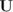and pressure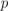, hence the entries for U and p. The keyword is followed by a dictionary containing the type of solver and the parameters that the solver uses. The solver is selected through the solver keyword from the choice in OpenFOAM, listed in Table 6.9. The parameters, including tolerance, relTol, preconditioner, etc. are described in following sections.

 Solver Keyword Preconditioned (bi-)conjugate gradient PCG/PBiCG† Stabilized Preconditioned (bi-)conjugate gradient (recommended over PCG/PBiCG) PBiCGStab Solver using a smoother smoothSolver Generalised geometric-algebraic multi-grid GAMG Diagonal solver for explicit systems diagonal †PCG for symmetric matrices, PBiCG for asymmetric

Table 6.9: Linear solvers.

The solvers distinguish between symmetric matrices and asymmetric matrices. The symmetry of the matrix depends on the structure of the equation being solved and, while the user may be able to determine this, it is not essential since OpenFOAM will produce an error message to advise the user if an inappropriate solver has been selected, e.g.

--> FOAM FATAL IO ERROR : Unknown asymmetric matrix solver PCG
Valid asymmetric matrix solvers are :
3
(
PBiCG
PBiCGStab
smoothSolver
GAMG
)

#### 6.3.1.1 Solution tolerances

The sparse matrix solvers are iterative, i.e. they are based on reducing the equation residual over a succession of solutions. The residual is ostensibly a measure of the error in the solution so that the smaller it is, the more accurate the solution. More precisely, the residual is evaluated by substituting the current solution into the equation and taking the magnitude of the difference between the left and right hand sides; it is also normalised in to make it independent of the scale of problem being analysed.

Before solving an equation for a particular field, the initial residual is evaluated based on the current values of the field. After each solver iteration the residual is re-evaluated. The solver stops if either of the following conditions are reached:

• the residual falls below the solver tolerance, tolerance;
• the ratio of current to initial residuals falls below the solver relative tolerance, relTol;
• the number of iterations exceeds a maximum number of iterations, maxIter;

The solver tolerance should represent the level at which the residual is small enough that the solution can be deemed sufficiently accurate. The solver relative tolerance limits the relative improvement from initial to final solution. In transient simulations, it is usual to set the solver relative tolerance to 0 to force the solution to converge to the solver tolerance in each time step. The tolerances, tolerance and relTol must be specified in the dictionaries for all solvers; maxIter is optional.

#### 6.3.1.2 Preconditioned conjugate gradient solvers

There are a range of options for preconditioning of matrices in the conjugate gradient solvers, represented by the preconditioner keyword in the solver dictionary. The preconditioners are listed in Table 6.10.

 Preconditioner Keyword Diagonal incomplete-Cholesky (symmetric) DIC Faster diagonal incomplete-Cholesky (DIC with caching) FDIC Diagonal incomplete-LU (asymmetric) DILU Diagonal diagonal Geometric-algebraic multi-grid GAMG No preconditioning none

Table 6.10: Preconditioner options.

#### 6.3.1.3 Smooth solvers

The solvers that use a smoother require the smoother to be specified. The smoother options are listed in Table 6.11. Generally GaussSeidel is the most reliable option, but for bad matrices DIC can offer better convergence. In some cases, additional post-smoothing using GaussSeidel is further beneficial, i.e. the method denoted as DICGaussSeidel

 Smoother Keyword Gauss-Seidel GaussSeidel Diagonal incomplete-Cholesky (symmetric) DIC Diagonal incomplete-Cholesky with Gauss-Seidel (symmetric) DICGaussSeidel

Table 6.11: Smoother options.

The user must also specify the number of sweeps, by the nSweeps keyword, before the residual is recalculated, following the tolerance parameters.

#### 6.3.1.4 Geometric-algebraic multi-grid solvers

The generalised method of geometric-algebraic multi-grid (GAMG) uses the principle of: generating a quick solution on a mesh with a small number of cells; mapping this solution onto a finer mesh; using it as an initial guess to obtain an accurate solution on the fine mesh. GAMG is faster than standard methods when the increase in speed by solving first on coarser meshes outweighs the additional costs of mesh refinement and mapping of field data. In practice, GAMG starts with the mesh specified by the user and coarsens/refines the mesh in stages. The user is only required to specify an approximate mesh size at the most coarse level in terms of the number of cells nCoarsestCells.

The agglomeration of cells is performed by the algorithm specified by the agglomerator keyword. Presently we recommend the faceAreaPair method. It is worth noting there is an MGridGen option that requires an additional entry specifying the shared object library for MGridGen:

geometricGamgAgglomerationLibs ("libMGridGenGamgAgglomeration.so");
In the experience of OpenCFD, the MGridGen method offers no obvious benefit over the faceAreaPair method. For all methods, agglomeration can be optionally cached by the cacheAgglomeration switch.

Smoothing is specified by the smoother as described in section 6.3.1.3. The number of sweeps used by the smoother at different levels of mesh density are specified by the nPreSweeps, nPostSweeps and nFinestSweeps keywords. The nPreSweeps entry is used as the algorithm is coarsening the mesh, nPostSweeps is used as the algorithm is refining, and nFinestSweeps is used when the solution is at its finest level.

The mergeLevels keyword controls the speed at which coarsening or refinement levels is performed. It is often best to do so only at one level at a time, i.e. set mergeLevels 1. In some cases, particularly for simple meshes, the solution can be safely speeded up by coarsening/refining two levels at a time, i.e. setting mergeLevels 2.

### 6.3.2 Solution under-relaxation

A second sub-dictionary of fvSolution that is often used in OpenFOAM is relaxationFactors which controls under-relaxation, a technique used for improving stability of a computation, particularly in solving steady-state problems. Under-relaxation works by limiting the amount which a variable changes from one iteration to the next, either by modifying the solution matrix and source prior to solving for a field or by modifying the field directly. An under-relaxation factor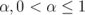specifies the amount of under-relaxation, ranging from none at all for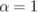and increasing in strength as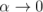. The limiting case where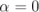represents a solution which does not change at all with successive iterations. An optimum choice of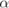is one that is small enough to ensure stable computation but large enough to move the iterative process forward quickly; values ofas high as 0.9 can ensure stability in some cases and anything much below, say, 0.2 are prohibitively restrictive in slowing the iterative process.

OpenFOAM includes two variants of the SIMPLE algorithm, standard SIMPLE and its consistent formulation, SIMPLEC. By default SIMPLE is used. To use SIMPLEC, the switch

consistent      yes;
must be set in the SIMPLE subdirectory of the fvSolution dictionary The SIMPLEC formulation for the pressure-velocity coupling method needs only a small amount of under-relaxation for velocity and other transport equations. There is no need to use any relaxation on pressure. This results typically in more robust solution and faster convergence.

The user can specify the relaxation factor for a particular field by specifying first the word associated with the field, then the factor. The user can view the relaxation factors used in a tutorial example of simpleFoam for incompressible, laminar, steady-state flows.

17
18solvers
19{
20    p
21    {
22        solver          GAMG;
23        tolerance       1e-06;
24        relTol          0.1;
25        smoother        GaussSeidel;
26    }
27
28    "(U|k|epsilon|omega|f|v2)"
29    {
30        solver          smoothSolver;
31        smoother        symGaussSeidel;
32        tolerance       1e-05;
33        relTol          0.1;
34    }
35}
36
37SIMPLE
38{
39    nNonOrthogonalCorrectors 0;
40    consistent      yes;
41
42    residualControl
43    {
44        p               1e-2;
45        U               1e-3;
46        "(k|epsilon|omega|f|v2)" 1e-3;
47    }
48}
49
50relaxationFactors
51{
52    equations
53    {
54        U               0.9; // 0.9 is more stable but 0.95 more convergent
55        ".*"            0.9; // 0.9 is more stable but 0.95 more convergent
56    }
57}
58
59
60// ************************************************************************* //

### 6.3.3 PISO and SIMPLE algorithms

Most fluid dynamics solver applications in OpenFOAM use the pressure-implicit split-operator (PISO) or semi-implicit method for pressure-linked equations (SIMPLE) algorithms. These algorithms are iterative procedures for solving equations for velocity and pressure, PISO being used for transient problems and SIMPLE for steady-state.

Both algorithms are based on evaluating some initial solutions and then correcting them. SIMPLE only makes 1 correction whereas PISO requires more than 1, but typically not more than 4. The user must therefore specify the number of correctors in the PISO dictionary by the nCorrectors keyword as shown in the example on page 227.

An additional correction to account for mesh non-orthogonality is available in both SIMPLE and PISO in the standard OpenFOAM solver applications. A mesh is orthogonal if, for each face within it, the face normal is parallel to the vector between the centres of the cells that the face connects, e.g. a mesh of hexahedral cells whose faces are aligned with a Cartesian coordinate system. The number of non-orthogonal correctors is specified by the nNonOrthogonalCorrectors keyword as shown in the examples above and on page 227. The number of non-orthogonal correctors should correspond to the mesh for the case being solved, i.e. 0 for an orthogonal mesh and increasing with the degree of non-orthogonality up to, say, 20 for the most non-orthogonal meshes.

#### 6.3.3.1 Pressure referencing

In a closed incompressible system, pressure is relative: it is the pressure range that matters not the absolute values. In these cases, the solver sets a reference level of pRefValue in cell pRefCell where p is the name of the pressure solution variable. Where the pressure is p_rgh, the names are p_rhgRefValue and p_rhgRefCell respectively. These entries are generally stored in the PISO/SIMPLE sub-dictionary and are used by those solvers that require them when the case demands it. If omitted, the solver will not run, but give a message to alert the user to the problem.

### 6.3.4 Other parameters

The fvSolutions dictionaries in the majority of standard OpenFOAM solver applications contain no other entries than those described so far in this section. However, in general the fvSolution dictionary may contain any parameters to control the solvers, algorithms, or in fact anything. For a given solver, the user can look at the source code to find the parameters required. Ultimately, if any parameter or sub-dictionary is missing when an solver is run, it will terminate, printing a detailed error message. The user can then add missing parameters accordingly.